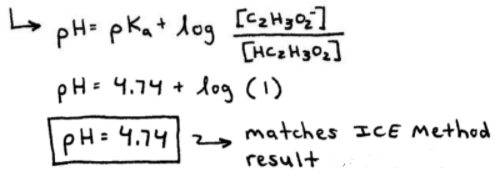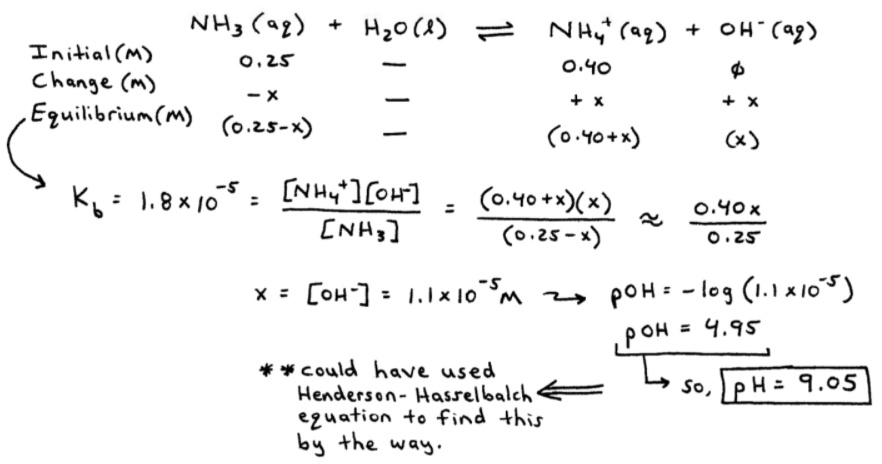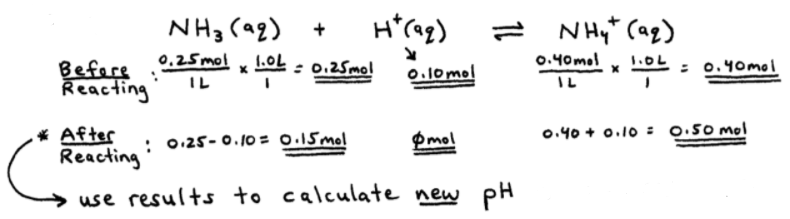# S15E3 - The Henderson-Hasselbalch Equation

In a buffer solution, the pH is governed by the ratio of [HA-] (weak acid) to the common ion, [A-].

The pH depends on the ratio [HA-] / [A-] and for problem-solving, this ratio is integrated into the Henderson-Hasselbalch Equation.

## Henderson-Hasselbalch Equation

### pH  =  pKa  +  log ( [A-] / [HA-] )

➞  note:  pKa = - log Ka

➞  this is a quick and easy way to replace ICE Tables for buffer calculations.----------

ex:  Using the Henderson-Hasselbalch equation, calculate the pH of a buffer solution containing 0.50 M HC2H3O2 and 0.50 M NaC2H3O2.  The Ka for acetic acid is 1.8 x 10-5.
_________

First let's use the given Ka value to find the pKa...

pKa  =  - log Ka

pKa  =  - log ( 1.8 x 10-5)

pKa  =  4.74

Now, we'll use the pKa value in the Henderson-Hasselbalch equation to solve for the pH of the buffer:

pH  =  pKa  +  log ( [C2H3O2-] / [HC2H3O2] )

pH  =  4.74  +  log ( 0.50 M / 0.50 M )

pH  =  4.74  +  log ( 1 )

pH  =  4.74  ➞  matches ICE Table result from the previous video.

➞  see the image below for a better view of our set-up...----------

ex A buffer solution contains 0.25 M NH3 (Kb = 1.8 x 10-5) and 0.40 M NH4Cl.  Calculate the pH.
_________

Alright, our common ion is NH4+

- major species:  NH3H2O (neutral) , NH4+ , Cl- (neutral)

- so we have:  let's use an ICE Table (yes, we could use the H-H Equation here)----------

Now, what would be the pH IF 0.10 mol HCl were added to 1.0 L of this buffer (above)?

before any reaction occurs:

- major species:  NH3  ,  NH4+  ,  H+ , Cl- (neutral) , H2O (neutral)

➞ so NH3(aq) will react with H+(aq) as shown below:See the "after results" of 0.15 mol NH3 and 0.50 mol NH4+?  We can use them in another ICE Table or the Henderson-Hasselbalch equation.

Let's use the H-H equation...==========

## Titrations and Titration Curves

Titration Curves = also called "pH Curves."

Titrations are performed to determine the amount of acid or base in a solution.

A buret and an erlenmeyer flask are used, as shown in the image below...As you can see, the buret contains the titrant (the acid or base) - whichever one we know more about.

In other words, the buret contains the "known" solution.

The acid or base titrant solution has both a known concentration (molarity, M) and a known volume delivered (mL) to the erlenmeyer flask.

The erlenmeyer flask contains the "unknown" analyte solution, where we know the volume (mL) but not the concentration (molarity, M).

If the analyte is a base, the titrant will be an acid -- or vice-versa.

----------

### Acid-Base Titrations

We will do a zillion examples in the videos that follow, but I want to make two more points about Acid-Base Titrations before we move on:

1.  At the stoichiometric point of the acid-base reaction (the equivalence point of the titration), moles of acid equals moles of base.

In other words, the total # of moles of acid equals the total # of moles of base:

➞    Moles of ACID   =   Moles of BASE

2.  The progress of an an acid-base titration can be monitored by plotting the pH (y-axis) vs. the amount of titrant added (x-axis).

➞    this plot is called a titration curve (ph curve).

----------

### 2 Types of Acid-Base Titrations

i.
Strong Acid - Strong Base Titrations

- similarly, Strong Base - Strong Acid Titrations

ii.  Weak Acid - Strong Base Titrations

- similarly, Weak Base - Strong Acid Titrations

==========

In the next few videos in SECTION 15 - Applications of Acid-Base Equilibria,

We'll go over a ton of acid-base titration examples and then graph the titration curves...